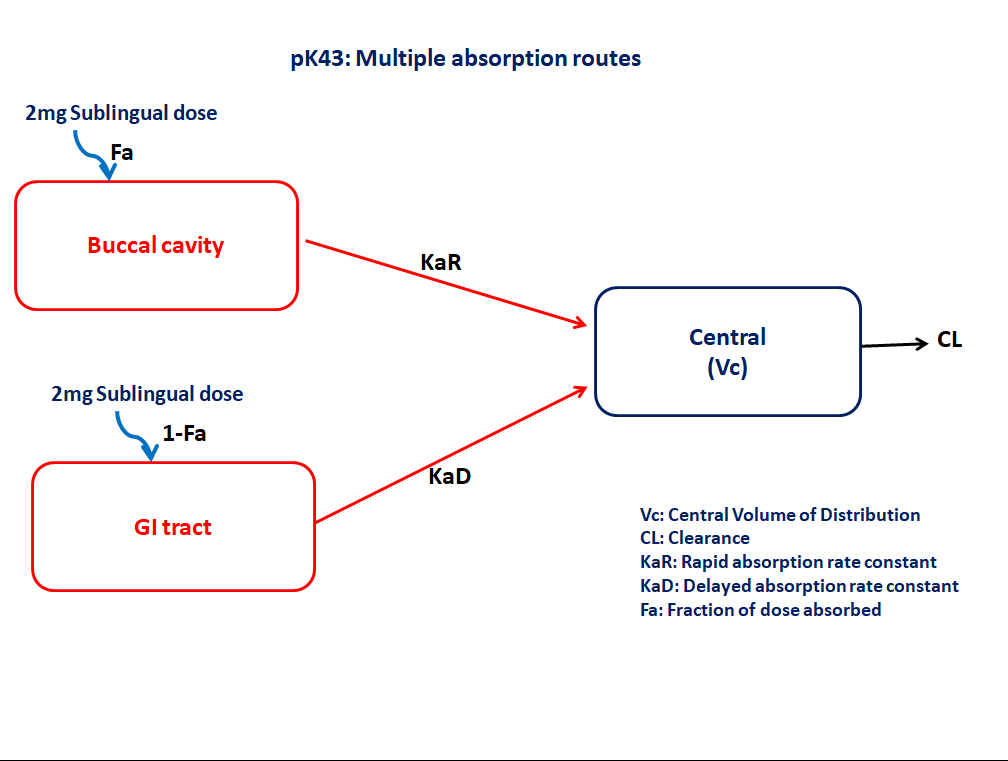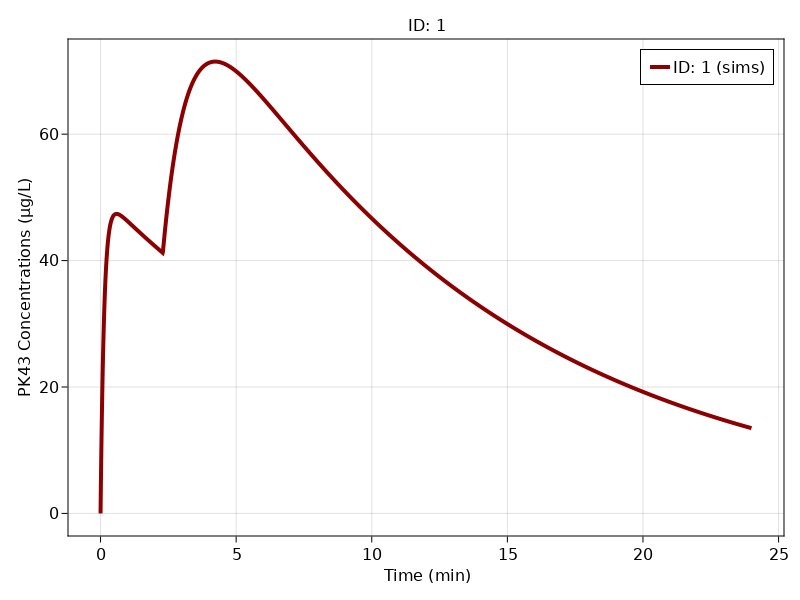# Exercise PK43 - Multiple absorption routes

### Background

• Structural model - 1 compartment linear elimination with first order absorption

• Route of administration - Sublingual

• Dosage Regimen - 2mg Sublingual dose

• Number of Subjects - 1### Learning Outcome

In this model, Multiple absorption routes helps in understanding absorption of sublingually administered dose partly from buccal cavity and partly from gastrointestinal tract

### Objectives

In this model you will learn how to write differential equation model for a drug that is partly absorbed from buccal and partly from GI and simulate for a single subject.

### Libraries

Call the "necessary" libraries to get start.

using Random
using Pumas
using PumasUtilities
using CairoMakie


### Model

In this one compartment model, we administer dose Sublingually.

pk_43        = @model begin
desc     = "Multiple Absorption Model"
timeu    = u"hr"
end

@param begin
"Absorption rate constant(rapid from buccal) (hr⁻⅟)"
tvkar    ∈ RealDomain(lower=0)
"Absorption rate constant(delayed from GI) (hr⁻⅟)"
"Volume of Central Compartment (L)"
tvv      ∈ RealDomain(lower=0)
"Lagtime (hr)"
tvlag    ∈ RealDomain(lower=0)
"Fraction of drug absorbed"
tvfa     ∈ RealDomain(lower=0)
"Elimination rate constant (hr⁻⅟)"
tvk      ∈ RealDomain(lower=0)
Ω        ∈ PDiagDomain(4)
end

@random begin
η        ~ MvNormal(Ω)
end

@pre begin
KaR      = tvkar * exp(η)
V        = tvv * exp(η)
K        = tvk * exp(η)
end

@dosecontrol begin
bioav    = (Buccal=tvfa, Gi= 1-tvfa)
lags     = (Gi=tvlag,)
end

@dynamics begin
Buccal'  = -KaR*Buccal
Central' =  KaR*Buccal + KaD*Gi - K*Central
end

@derived begin
cp       = @. Central/V
"""
Observed Concentration (mcg/L)
"""
end
end

PumasModel
Random effects: η
Covariates:
Dynamical variables: Buccal, Gi, Central
Derived: cp, dv
Observed: cp, dv


### Parameters

Parameters provided for simulation. tv represents the typical value for parameters.

• $Vc$ - Volume of Central Compartment (L)

• $K$ - Elimination rate constant (hr⁻⅟)

• $Kar$ - Absorption rate constant(rapid from buccal) (hr⁻⅟)

• $Kad$ - Absorption rate constant(delayed from GI) (hr⁻⅟)

• $Fa$ - Fraction of drug absorbed

• $lags$ - Lagtime (hr)

• $Ω$ - Between Subject Variability

• $σ$ - Residual error

param = (tvkar  = 7.62369,
tvv    = 20.6274,
tvk    = 0.0886931,
tvfa   = 0.515023,
tvlag  = 2.29614,
Ω      = Diagonal([0.0,0.0,0.0,0.0]),

(tvkar = 7.62369, tvkad = 1.0751, tvv = 20.6274, tvk = 0.0886931, tvfa = 0.
515023, tvlag = 2.29614, Ω = [0.0 0.0 0.0 0.0; 0.0 0.0 0.0 0.0; 0.0 0.0 0.0
0.0; 0.0 0.0 0.0 0.0], σ_add = 0.86145)


### DosageRegimen

Single subject receiving 2mg dose given orally (absorbed - Sublingually and remaining from the Gut).

ev1  = DosageRegimen(2000, time = 0, cmt = [1,2])
sub1 = Subject(id = 1, events = ev1)

Subject
ID: 1
Events: 2


### Simulation

Lets simulate plasma concentration with specific observation times after Sublingual dose considering multiple absorption routes.

Random.seed!(123)
sim_sub1 = simobs(pk_43, sub1, param, obstimes=0.00:0.01:24)


### Visualization

f1, a1, p1 = sim_plot(pk_43, [sim_sub1],
observations = :cp,
color = :redsblues,
linewidth = 4,
axis = (
xlabel = "Time (min)",
ylabel = "PK43 Concentrations (μg/L)",
xticks = 0:5:25))
axislegend(a1)
f1